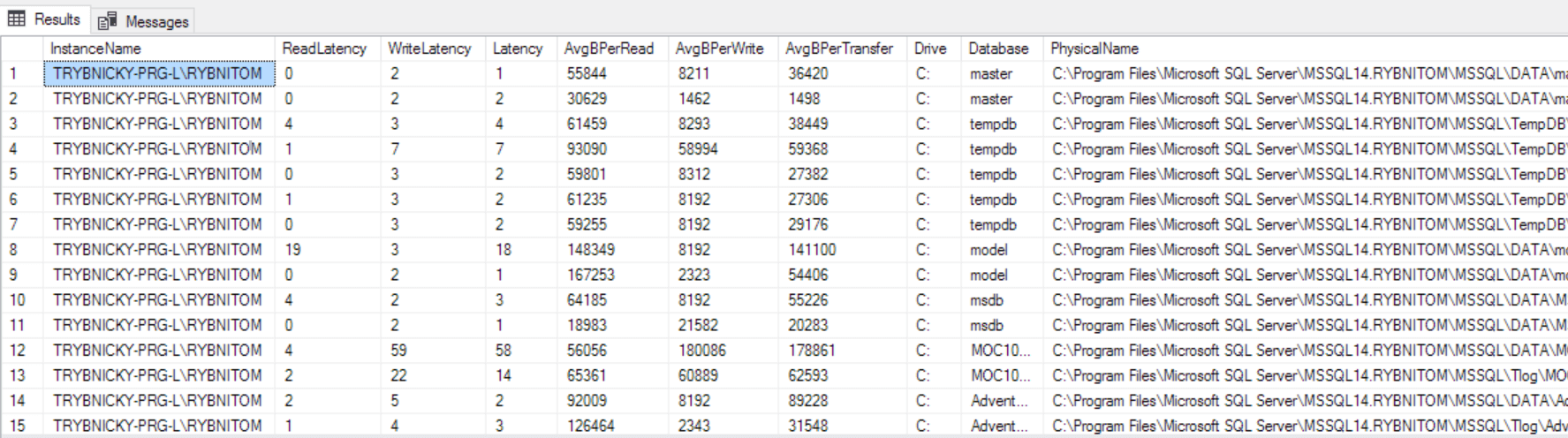# SQL Server - Data files latency

Examine performance of particular data files for all databases. Useful when troubleshooting performance of your SQL Server. Latency is one of the main performance counters measuring IO performance for SQL Server. It is well known that most common performance killers are IO bottlenecks pointing to slow disk drives.

TIP: Use commented filtering clause and sorting statements to find your troublemakers fast.

```SELECT
CONVERT(VARCHAR(250), SERVERPROPERTY('ServerName')) AS InstanceName
WHEN [num_of_reads] = 0 THEN 0
END
,[WriteLatency] = CASE
WHEN [num_of_writes] = 0 THEN 0
ELSE ([io_stall_write_ms] / [num_of_writes])
END
,[Latency] = CASE
WHEN ([num_of_reads] = 0 AND [num_of_writes] = 0) THEN 0
ELSE ([io_stall] / ([num_of_reads] + [num_of_writes]))
END
WHEN [num_of_reads] = 0 THEN 0
END
,[AvgBPerWrite] = CASE
WHEN [num_of_writes] = 0 THEN 0
ELSE ([num_of_bytes_written] / [num_of_writes])
END
,[AvgBPerTransfer] = CASE
WHEN ([num_of_reads] = 0 AND [num_of_writes] = 0) THEN 0
END
,LEFT([mf].[physical_name], 2) AS [Drive]
,DB_NAME([vfs].[database_id]) AS [DB]
,[mf].[physical_name]
FROM sys.dm_io_virtual_file_stats(NULL, NULL) AS [vfs]
INNER JOIN sys.master_files AS [mf] ON [vfs].[database_id] = [mf].[database_id] AND [vfs].[file_id] = [mf].[file_id]
-- WHERE [vfs].[file_id] = 2 -- log files
-- ORDER BY [Latency] DESC
-- ORDER BY [WriteLatency] DESC;
GO```

#### Results

Script results screen:Script results detailed description:

Column nameData typeDescription
InstanceNamevarchar(250)Name of actual SQL Server instance. Useful for multi-querying.